# When the Elevator Goes up with Acceleration 1.2 M/S2: When the Elevator Goes up with Acceleration 1.2 M/S2: - Physics

Sum

A pendulum bob of mass 50 g is suspended from the ceiling of an elevator. Find the tension in the string if the elevator (a) goes up with acceleration 1.2 m/s2, (b) goes up with deceleration 1.2 m/s2, (c) goes up with uniform velocity, (d) goes down with acceleration 1.2 m/s2, (e) goes down with deceleration 1.2 m/s2 and (f) goes down with uniform velocity.

#### Solution

(a) When the elevator goes up with acceleration 1.2 m/s2: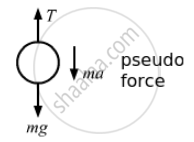$T = mg + ma$
⇒ T = 0.05 (9.8 + 1.2) = 0.55 N
(b) Goes up with deceleration 1.2 m/s2 :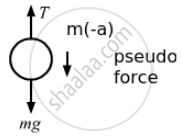$T = mg + m\left( - a \right) = m\left( g - a \right)$
⇒ T  = 0.05 (9.8 − 1.2) = 0.43 N

(c) Goes up with uniform velocity: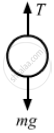$T = mg$
⇒ T = 0.05 × 9.8 = 0.49 N

(d) Goes down with acceleration 1.2 m/s2 :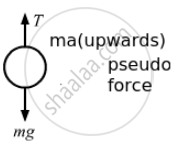$T + ma = mg$
$\Rightarrow T = m\left( g - a \right)$
⇒ T = 0.05 (9.8 − 1.2) = 0.43 N

(e) Goes down with deceleration 1.2 m/s2 :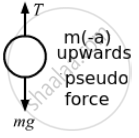$T + m\left( - a \right) = mg$
$\Rightarrow T = m\left( g + a \right)$
⇒ = 0.05 (9.8 + 1.2) = 0.55 N

(f) Goes down with uniform velocity: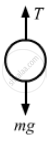$T = mg$
T = 0.05 × 9.8 = 0.49 N

Concept: Newton's Third Law of Motion
Is there an error in this question or solution?

#### APPEARS IN

HC Verma Class 11, Class 12 Concepts of Physics Vol. 1
Chapter 5 Newton's Laws of Motion
Q 14 | Page 80